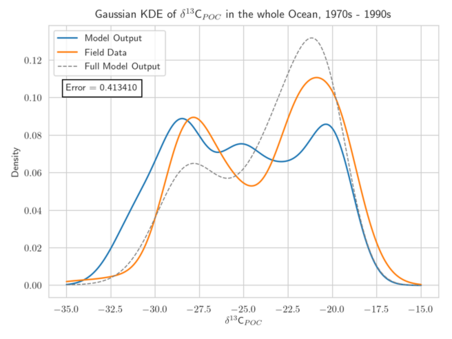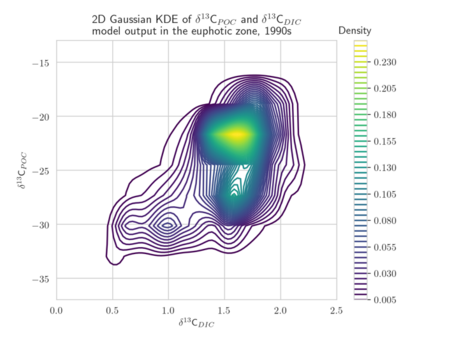# Calibration of marine biogeochemical models

## Development of adapted Kernel Density Estimators for the calibration of marine biogeochemical models

Doctoral Researcher:

Maria-Theresia Pelz, GEOMAR - Helmholtz Centre for Ocean Research Kiel, mverwega@geomar.de

Supervisors:

Location: Kiel

Disciplines: applied mathematics, numerical mathematics, statistics, biological oceanography

Keywords: data analysis, data-model comparison, parameter estimationExample of a one-dimensional Gaussian Kernel Density Estimators for isotopic data.

Motivation: The assessment and calibration of climate models relies on the comparison of model output and available data. In model assessment, the model-data-misfit is used as a quality criterion to compare different models. In model calibration, a mathematical optimization procedure is applied to “tune” the models, i.e. their parameters, to obtain the best possible model-data fit. In both applications, the choice of the measure, criterion or metric is crucial and influences the results. In model calibration, it additionally determines the efficiency and accuracy of the optimization algorithm. In many cases, least-squares type metrics are used. If they include covariance information, the result of an optimization run can be interpreted as a maximum-likelihood estimator, assuming a Gaussian distribution of the data. In the context of marine isotopic data, a non-parametric statistical approach based on Kernel Density Estimators (KDEs) is assumed to be superior to point-by-point root-mean-square error (Somes et al. 2017). KDEs allow for a statistical analysis without prescribing a probability density. KDEs are well suited for comparing sparse observations with large data sets and for the evaluation of large numbers of model results (e.g. multi-model ensembles).Example of a two-dimensional Gaussian Kernel Density Estimators for isotopic data.

Aim: The project focuses on the development of adapted KDEs for model and observational data, computed as solution of a diffusion-type parabolic equation with appropriate coefficient functions (Botev et al. 2010). These KDEs are a generalization of the well-established Gaussian KDEs (see figure). They avoid the typical Gaussian boundary bias for bounded data sets and include adapted and optimized smoothing properties. The target is to develop KDEs which are best suited for one- and multi-dimensional marine biogeochemical data. A further aim is the determination of the corresponding optimal bandwidth. The diffusion-based KDEs shall be evaluated using different metrics for model calibration/data assimilation of exemplary global biogeochemical models (e.g., Schmittner Somes 2016). We will use the simulation and optimization software Metos3D (Piwonski Slawig 2016) as well as given 13C and 15N isotopic data. The solution of the underlying diffusion-type equation shall be computed numerically. Different optimization algorithms provided by Metos3D shall be applied and compared. The project shall provide a valuable contribution to assessing parameter uncertainties to climate states and to improved predictions of marine biogeochemical cycling.

Objectives: (1) Mathematical analysis of diffusion-based KDEs for the application on climate model output and data. (2) Implementation of a software framework for diffusion-based KDEs. (3) Exemplary application on given marine biogeochemical models and isotopic data.

References:

• Botev, Z.I., Grotowski, J.F., Kroese, D.P. (2010): Kernel density estimation by diffusion, Annals of Statistics 38(5).
• Piwonski, J., Slawig, T. (2016): Metos3D: The Marine Ecosystem Toolkit for Optimization and Simulation in 3-D - Part 1: Simulation Package v0.3.2, Geoscientific Model Development, 9, 3729-3750.
• Schmittner, A., Somes, C. J. (2016): Complementary constraints from carbon (13C) and nitrogen (15N) isotopes on the glacial ocean’s soft-tissue biological pump, Paleoceanography, 31, 669–693.
• Somes, C. J. , Schmittner, A., Muglia, J., Oschlies, A. (2017): A three-dimensional model of the marine nitrogen cycle during the last glacial maximum constrained by sedimentary isotopes. Frontiers in Marine Science, 4:108.